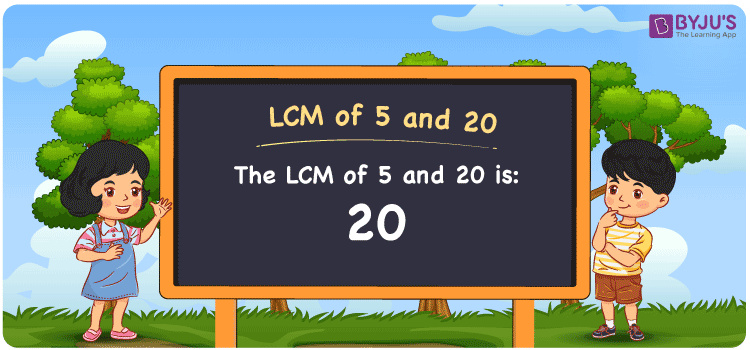Checkout JEE MAINS 2022 Question Paper Analysis : Checkout JEE MAINS 2022 Question Paper Analysis :

# LCM of 5 and 20

LCM of 5 and 20 is 20. The LCM value is the number divisible evenly by the given numbers 5 and 20. The common multiples among the two numbers will help you find the least common multiple of 5 and 20. The multiples of 5 are (5, 10, 15, 20, 25, 30, ….) and the multiples of 20 are (20, 40, 60, 80, 100, ….) respectively. You can find the LCM using prime factorisation method, division method and by listing out the multiples of 5 and 20.

## What is LCM of 5 and 20?

The answer to this question is 20. The LCM of 5 and 20 using various methods is shown in this article for your reference. The LCM of two non-zero integers, 5 and 20, is the smallest positive integer 20 which is divisible by both 5 and 20 with no remainder.## How to Find LCM of 5 and 20?

LCM of 5 and 20 can be found using three methods:

• Prime Factorisation
• Division method
• Listing the multiples

### LCM of 5 and 20 Using Prime Factorisation Method

The prime factorisation of 5 and 20, respectively, is given by:

5 = 5¹

20 = 2 x 2 x 5 = 2² x 5¹

LCM (5, 20) = 20

### LCM of 5 and 20 Using Division Method

We’ll divide the numbers (5, 20) by their prime factors to get the LCM of 5 and 20 using the division method (preferably common). The LCM of 5 and 20 is calculated by multiplying these divisors.

 2 5 20 2 5 10 5 1 5 x 1 1

No further division can be done.

Hence, LCM (5, 20) = 20

### LCM of 5 and 20 Using Listing the Multiples

To calculate the LCM of 5 and 20 by listing out the common multiples, list the multiples as shown below

 Multiples of 5 Multiples of 20 5 20 10 40 15 60 20 80 25 100 30 120 35 140

LCM (5, 20) = 20

## Video Lesson on Applications of LCM## LCM of 5 and 20 Solved Examples

The LCM and GCD of two numbers are 20 and 5 respectively. If one number is 20, what is the other number?

Solution:

Consider the number as z

We know that

GCD x LCM = 20 x z

z = (GCD x LCM)/20

Substituting the values

z = (20 x 5)/20

z = 5

Hence, the other number is 5.

## Frequently Asked Questions on LCM of 5 and 20

### Calculate the GCF if the LCM of 5 and 20 is 20.

We know that;

LCM x GCF = 5 x 20

As LCM (5, 20) is 20

20 x GCF = 100

GCF = 100/20 = 5

### What is the LCM of 5 and 20?

The LCM of 5 and 20 is 20.We first should know the multiples of 5 and 20 in order to find the LCM

Multiples of 5 = 5, 10, 15, 20, 25, 30, ……

Multiples of 20 = 20, 40, 60, 80, 100, 120 ….

The smallest multiple which is exactly divisible by 5 and 20 is 20.

### Show the relation between GCF and LCM of 5 and 20.

The relation between GCF and LCM of 5 and 20 is

LCM x GCF = 5 x 20

LCM x GCF = 100